# User:IssaRice/Type checking Pearl's belief propagation notation

this is all from Judea Pearl's Probabilistic Reasoning in Intelligent Systems, chapter 4.

## Probability of a random variable

Pearl writes expressions like "$\lambda(x)$" and "$\pi(x)$", but I think these can't be understood literally. If$x$ is in the range of a random variable$X$, then we should be able to write things like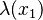$\lambda(x_1)$ as well. But$\lambda(x)$ is actually a vector standing for all possible values in the range of$X$.

My own attempt at making this more coherent is the following. Let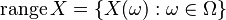$\operatorname{range} X = \{X(\omega) : \omega \in \Omega\}$, and let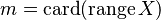$m = \operatorname{card} (\operatorname{range} X)$. In other words,$n$ is the number of distinct values that$X$ takes on. Then we define the vector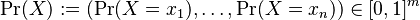$\Pr(X) := (\Pr(X = x_1), \ldots, \Pr(X = x_n)) \in [0,1]^m$

where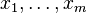$x_1, \ldots, x_m$ are the$m$ distinct values of$X$. A small problem is that we have to fix some ordering of$x_1, \ldots, x_m$. Since$x_1, \ldots, x_m \in \mathbf R$, I think we can just pick indexes such that$x_1 < \cdots < x_m$.

Notice that we have defined the probability of a random variable (rather than a probability of an event).

We will also define the probability of a random variable conditioned on another random variable. This will be a matrix.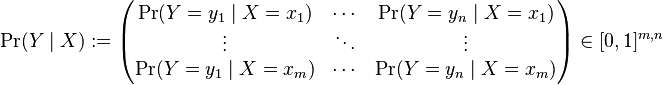$\Pr(Y\mid X) := \begin{pmatrix} \Pr(Y=y_1 \mid X=x_1) & \cdots &\Pr(Y=y_n \mid X=x_1) \\ \vdots & \ddots & \vdots \\ \Pr(Y=y_1 \mid X=x_m) & \cdots & \Pr(Y=y_n\mid X=x_m)\end{pmatrix} \in [0,1]^{m,n}$

where$m = \operatorname{card} (\operatorname{range} X)$ and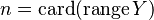$n = \operatorname{card} (\operatorname{range} Y)$.

Pearl writes the above matrix as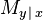$M_{y\,\vert\,x}$. How can you remember which way is x and which way is y? Pearl doesn't give any mnemonic for this, and I haven't thought of one yet. But this might not matter anyway; you can take the Pearlpill and say that all the matrices and vectors automatically reorient themselves to multiply along the common index!

I think the main problem with Pearl's notation is that sometimes the lowercase letters represent "possible values (in the abstract) of the variable" and other times they represent specific values. For example he writes: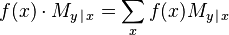$f(x) \cdot M_{y\,\vert\,x} = \sum_x f(x)M_{y\,\vert\,x}$

On the left side,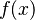$f(x)$ is a vector and$M_{y\,\vert\,x}$ is a matrix (representing the abstract possibilities), while on the right side,$f(x)$ is a number and$M_{y\,\vert\,x}$ is a vector (representing specific values)! The result is that you can't just look at something like "$M_{y\,\vert\,x}$" in isolation and know its type; you must always keep in mind the context. (This is similar to how in linear algebra,$0$ can be a scalar or vector of any size, depending on what the surrounding expressions are. The difference is that here, all the expressions are relativistic in this sense, so you don't have anything to ground you on.)

My own opinion is that the formalism of random variables takes care of representing "abstract possibilities", so why not just use that?

With the random variable notation, we can write: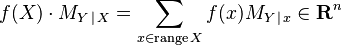$f(X) \cdot M_{Y\,\vert\,X} = \sum_{x\in\operatorname{range}X} f(x)M_{Y\,\vert\,x} \in \mathbf R^n$

where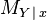$M_{Y\,\vert\,x}$ means the row of$M_{Y\,\vert\,X}$ where$X=x$.

A particularly confusing equation is 4.44: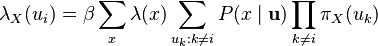$\lambda_X(u_i) = \beta \sum_x \lambda(x) \sum_{u_k:k\ne i} P(x\mid \mathbf u) \prod_{k\ne i} \pi_X(u_k)$

Some notes:

• What is the dimension? here$u_i$ is treated as an abstract variable, so it is a vector with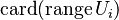$\operatorname{card}(\operatorname{range} U_i)$ components.
• What is the dimension of$\lambda(x)$? because of the summation over x, this is actually a real number.
• the$\mathbf u$ means that all of the "u"s are there, even$u_i$. but because the summation is taken over values of the "u"s other than$u_i$, this means that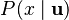$P(x\mid \mathbf u)$ is actually a vector with$\operatorname{card}(\operatorname{range} U_i)$ components. This is how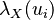$\lambda_X(u_i)$ gets its dimension.
• Each of the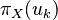$\pi_X(u_k)$s is a real number, because we are taking a summation over them. Indeed, if they were vectors, they would all potentially have a different dimension, so we wouldn't even be able to do elementwise multiplication.
• You might think it odd that we are taking a summation over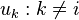$u_k : k\ne i$ and then taking a product over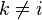$k\ne i$; aren't we bounding k twice?

I would write this as: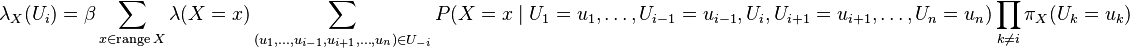$\lambda_X(U_i) = \beta \sum_{x\in\operatorname{range} X} \lambda(X=x) \sum_{(u_1,\ldots,u_{i-1},u_{i+1},\ldots,u_n)\in U_{-i}} P(X=x\mid U_1=u_1,\ldots,U_{i-1}=u_{i-1}, U_i, U_{i+1}=u_{i+1},\ldots,U_n=u_n) \prod_{k\ne i} \pi_X(U_k=u_k)$

where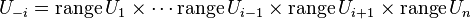$U_{-i} = \operatorname{range}U_1\times \cdots \operatorname{range}U_{i-1}\times \operatorname{range}U_{i+1}\times \operatorname{range}U_n$.

## Evidence

When Pearl writes evidence like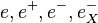$e, e^+, e^-, e^-_X$ and so on, I think in all cases these are events, i.e. subsets of the underlying sample space.

Therefore, expressions like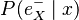$P(e^-_X\mid x)$ that involve a single "abstract" variable are vectors, in this case the vector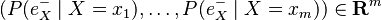$(P(e^-_X\mid X=x_1), \ldots, P(e^-_X\mid X=x_m)) \in \mathbf R^m$.

The evidence notation like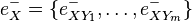$e^-_X = \{e^-_{XY_1},\ldots,e^-_{XY_m}\}$ is confusing to me. It is written like the elements of a set, and is also written as a disjunction, but seems to mean a conjunction (when written in probabilities, Pearl breaks them apart to multiply them).

For example on p. 165 he writes: "Thus, if X itself is not instantiated, we can write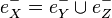$e_X^- = e_Y^- \cup e_Z^-$, and since X separates its children, we have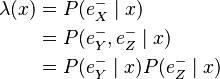\begin{align}\lambda(x) &= P(e_X^- \mid x) \\ &= P(e_Y^- , e_Z^- \mid x) \\ &= P(e_Y^-\mid x)P(e_Z^- \mid x)\end{align}

."

Here in "$e_X^- = e_Y^- \cup e_Z^-$" the nodes are being treated like sets containing the separate pieces of evidence, whereas in$P(e_Y^- , e_Z^- \mid x)$ the e's are being treated as events (the intersection of these events). Which looks odd because it violates the Leibniz law of substitution! We have to realize that "$e_X^-$" is being used in a different sense in the two expressions.

## Making sense of pi and lambda

When you see$\pi_Y(x)$ or$\lambda_X(u)$ how should you interpret this? In each case, the subscript is the child node, and the argument is the parent node. Thus,$X\to Y$ and$U\to X$. When you see$\pi$, that means a message from the parent to child, i.e. from X to Y. When you see$\lambda$, that means a message from the child to parent, i.e. from X to U.

Going the other direction, let's say we have the link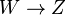$W\to Z$. What is the message from W to Z? It goes from parent to child, so must be a pi. And Z is the child, so takes the subscript. We get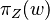$\pi_Z(w)$.

What is the message from Z to W? It goes from child to parent, so must be a lambda, and again Z takes the subscript, so we get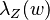$\lambda_Z(w)$.